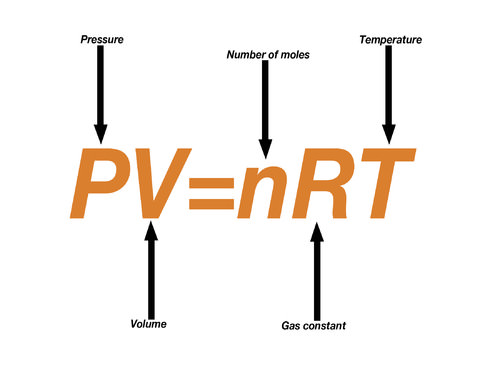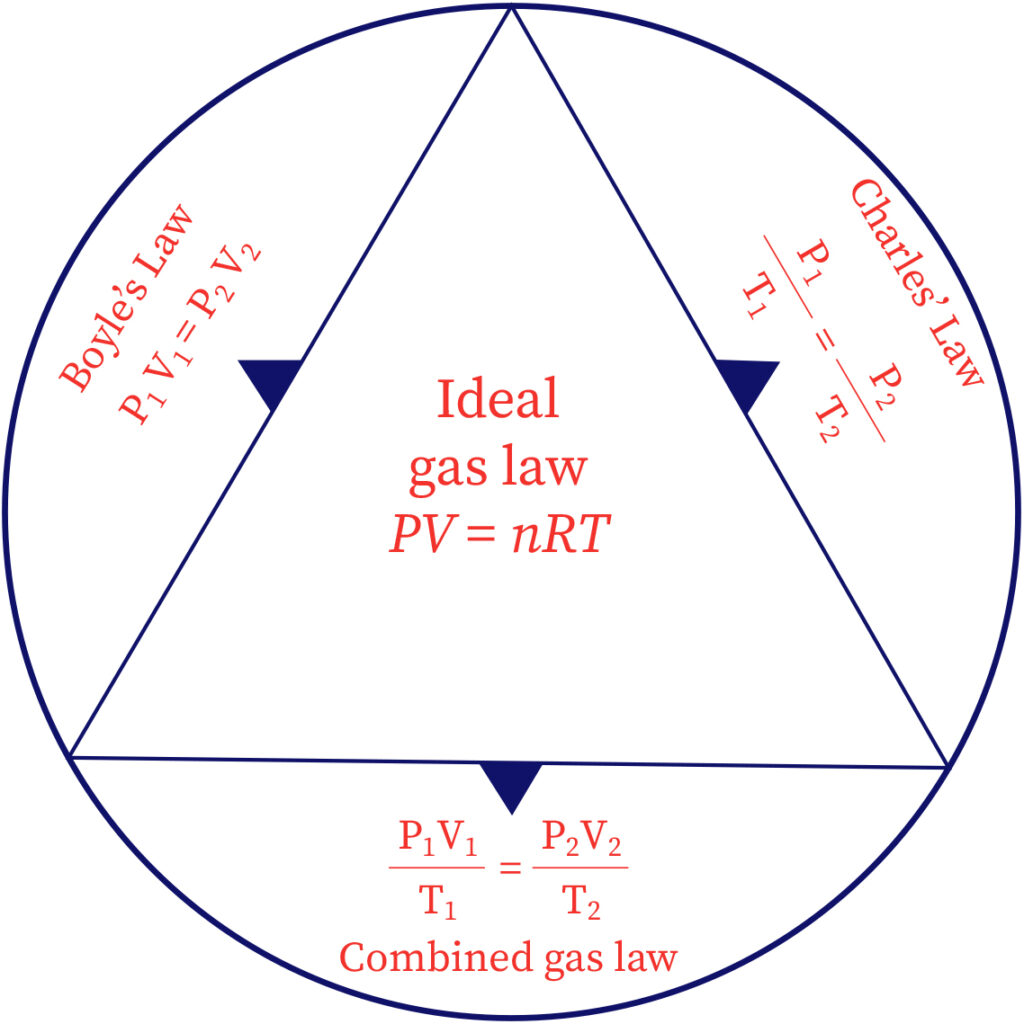# Combined Gas Law or the Gas Equation

• Combined gas law states that, for a given mass of a gas, the product of its pressure and volume divided by its Kelvin temperature is a constant.
• Boyle’s Law and Charle’s Law give separately the effect of pressure and temperature respectively on the volume of a gas.
• Combined Gas Law or the gas equation gives the simultaneous effect of the changes in pressure and temperature on the volume of a gas.
• It can be derived by combining Boyle’s Law and Charle’s Law as follows:

Let us suppose a certain quantity of a gas in initial state has volume V1 at temperature T1 and pressure P1. Let in final state the temperature and pressure be changed to T2 and P2 respectively, so that the volume changes to V2.

Further, suppose that this change is brought about in two steps as shown in figure. In the first step, suppose temperature is kept constant at T1, then, if the pressure is changed from P1 to P2, suppose volume changes from V1 to V.Image source: ck12

Applying Boyle’s Law, we have

P1V1 = P2V

V = P1V1/P2……………………………………… (i)

In the second step, suppose pressure is kept constant at P2. Now, if the temperature is changed from T1 to T2, the volume changes from V to V2.

Applying Charle’s Law, we have

V/T1  =  V2/T2…………………………………… (ii)

Substituting the value of V from equation (i) in equation (ii), we get

P1V1/P2T1=  V2/T2

Or, P1V1/T1  =  P2V2/T2………………….. (iii)

This implies that,

PV/T = a constant (say k)

Or, PV = kT………………………………………. (iv) where k is proportionality constant

Numerical value of proportionality constant depends upon the quantity of gas and the units in which volume and pressure are expressed but is totally independent of the nature of the gas.

For 1 mole of any gas, the constant ‘k’ is termed as the universal gas constant and is denoted by R

PV = RT………………………………………………. (v)

Equation (v) is known as the general gas equation or the ideal gas equation for one mole of gas.

For ‘n’ moles of a gas, therefore the gas equation may be written as

PV = n R T……………………………………………… (vi)Image source: bartleby                                        Image source: gaslaws

Nature of Gas Constant (R)

The exact nature of ‘R’ can be understood by considering the quantities involved in the gas equation.

From gas equation, PV = n R T, we have

R = PV/nT = pressure × volume/(number of moles × absolute temperature)

= force/area × volume/(no. of moles × absolute temperature)

= force/(length2) × (length3)/ (no. of moles × absolute temperature)

= (force × length)/(no. of moles ×absolute temperature)

= work/(no. of moles × absolute temperature)

Thus,’R’ represents work done per degree per mole. In CGS units it is expressed as ergs K-1 mole-1 and in SI units as Joule K-1mole-1.

References: